# MOS Interconnect and Crosstalk Using RFCKT Objects

This example shows how to build and simulate an RC tree circuit using the RF Toolbox™.

In "Asymptotic Waveform Evaluation for Timing Analysis" (IEEE Transactions on Computer-Aided Design, Vol., 9, No. 4, April 1990), Pillage and Rohrer presented and simulated an RC tree circuit that models signal integrity and crosstalk in low- to mid-frequency MOS circuit interconnect. This example confirms their simulations using RF Toolbox software.

Their circuit, reproduced in the following figure, consists of 11 resistors and 12 capacitors. In the paper, Pillage and Rohrer:

• Apply a ramp voltage input

• Compute transient responses

• Plot the output voltages across two different capacitors, `C7` and `C12`.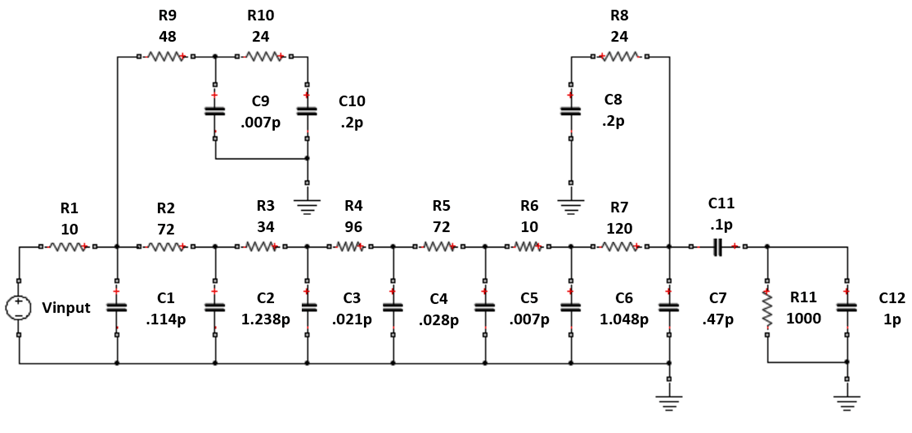Figure 1: RC tree model of MOS interconnect with crosstalk.

With RF Toolbox software, you can programmatically construct this circuit in MATLAB and perform signal integrity simulations.

This example shows:

1. How to use `rfckt.seriesrlc`, `rfckt.shuntrlc`, `rfckt.series`, and `rfckt.cascade` object to programmatically construct the circuit as two different networks, depending on the desired output.

2. How to use `analyze` function to extract the S-parameters for each 2-port network over a wide frequency range.

3. How to use `s2tf` function with `Zsource = 0` and `Zload = Inf` to compute the voltage transfer function from input to each desired output.

4. How to use `rationalfit` function to produce rational-function approximations that capture the ideal RC-circuit behavior to a very high degree of accuracy.

5. How to use `timeresp` function to compute the transient response to the input voltage waveform.

### Redraw Circuit as Distinct 2-Port Networks

To duplicate both output plots, RF Toolbox calculates the output voltage across `C7` and `C12`. To that end, the circuit must be expressed as two distinct 2-port networks, each with the appropriate capacitor at the output. Figure 2 shows the 2-port configuration for computing the voltage across `C7`. Figure 3 shows the configuration for `C12`. Both 2-port networks retain the original circuit topology, and share much of the same structure.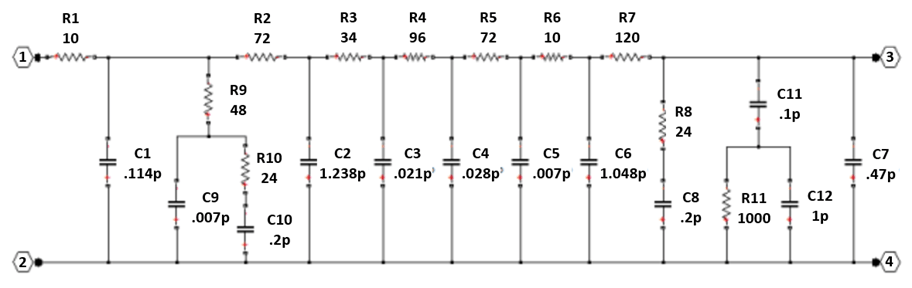Figure 2: The circuit drawn as a 2-port network with output across `C7`.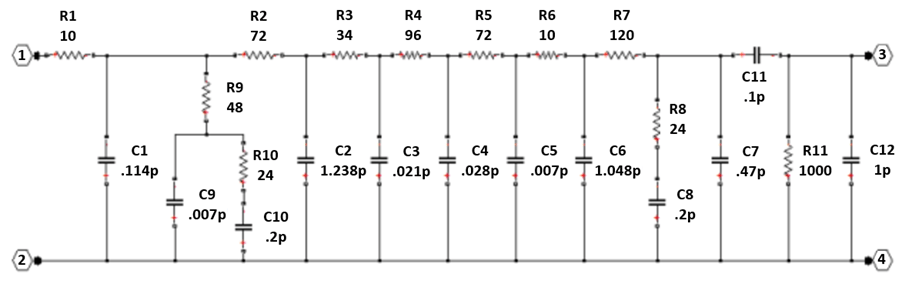Figure 3: The circuit drawn as a 2-port network with output across `C12`.

### Using RLC Building Blocks

All of the building blocks are formed by selecting appropriate values with the `rfckt.shuntrlc` object shown in Figure 4 or the `rfckt.seriesrlc` object shown in Figure 5. The 2-port building blocks are then connected using `rfckt.cascade` object as shown in Figure 6 or `rfckt.series` object as shown in Figure 7.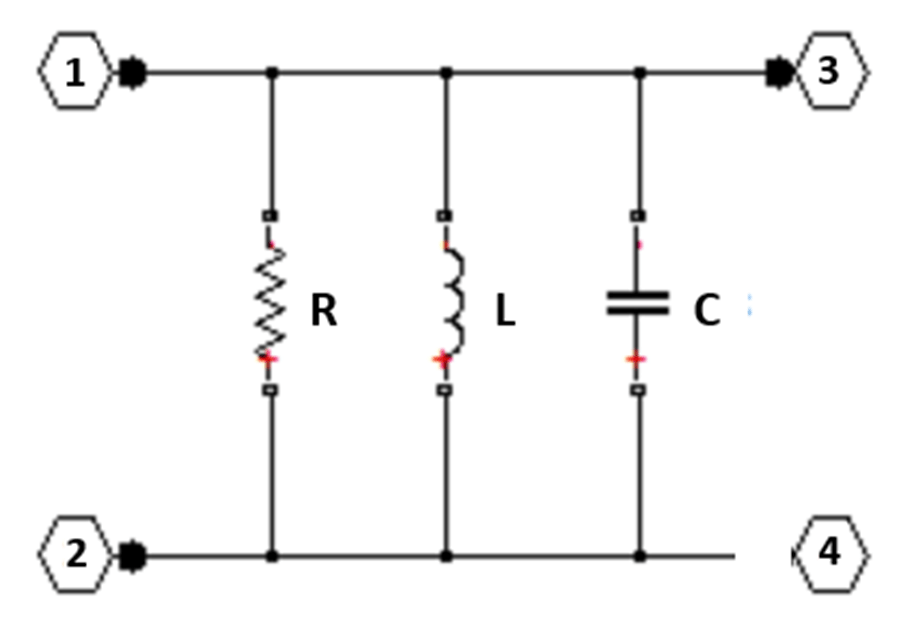Figure 4: The 2-port network created using the `rfckt.shuntrlc` object.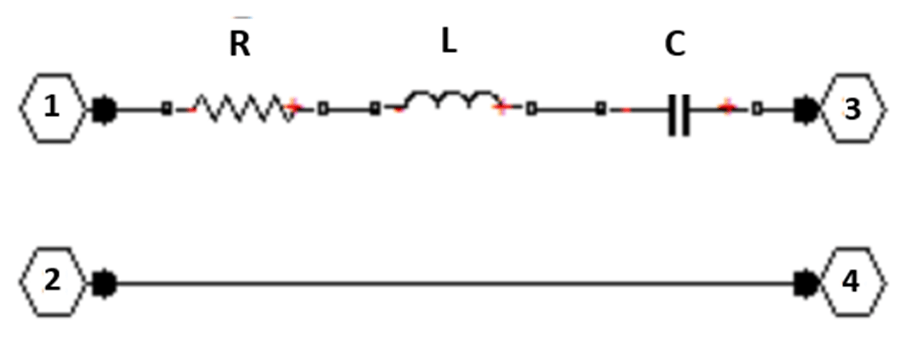Figure 5: The 2-port network created using the `rfckt.seriesrlc` object.Figure 6: Connect 2-port networks with the `rfckt.cascade` object.Figure 7: Connect 2-port networks with the `rfckt.series` object.

### Shared Pieces of 2-Port Networks

The following MATLAB code constructs the portion of the network shared between the two variants.

```R1 = rfckt.seriesrlc('R',10); C1 = rfckt.shuntrlc('C',0.114e-12); R9 = rfckt.shuntrlc('R',48); C9 = rfckt.shuntrlc('C',0.007e-12); R10 = rfckt.shuntrlc('R',24); C10 = rfckt.shuntrlc('C',0.2e-12); R10C10 = rfckt.series('Ckts',{R10,C10}); C9R10C10 = rfckt.cascade('Ckts',{C9,R10C10}); R9C9R10C10 = rfckt.series('Ckts',{R9,C9R10C10}); R2 = rfckt.seriesrlc('R',72); C2 = rfckt.shuntrlc('C',1.238e-12); R3 = rfckt.seriesrlc('R',34); C3 = rfckt.shuntrlc('C',0.021e-12); R4 = rfckt.seriesrlc('R',96); C4 = rfckt.shuntrlc('C',0.028e-12); R5 = rfckt.seriesrlc('R',72); C5 = rfckt.shuntrlc('C',0.007e-12); R6 = rfckt.seriesrlc('R',10); C6 = rfckt.shuntrlc('C',1.048e-12); R7 = rfckt.seriesrlc('R',120); R8 = rfckt.shuntrlc('R',24); C8 = rfckt.shuntrlc('C',0.2e-12); R8C8 = rfckt.series('Ckts',{R8,C8}); sharedckt = rfckt.cascade('Ckts', ... {R1,C1,R9C9R10C10,R2,C2,R3,C3,R4,C4,R5,C5,R6,C6,R7,R8C8}); % Additional shared building blocks used in both 2-port networks. C7 = rfckt.shuntrlc('C',0.47e-12); R11C12 = rfckt.shuntrlc('R',1000,'C',1e-12);```

### Construct Each 2-Port Network

Figure 2 shows that constructing a 2-port network with an output port across `C7` requires creating `C11` using `rfckt.shuntrlc` object, then combining `C11` with `R11` and `C12` using `rfckt.series` object, and finally combining `C11R11C12` with the rest of the network and `C7` using `rfckt.cascade` object.

Similarly, Figure 3 shows that constructing a 2-port network with an output port across `C12` requires creating another version of `C11` (`C11b`) using `rfckt.seriesrlc` object and combining all the parts together using `rfckt.cascade` object.

Construct shunt RLC circuit.

```C11 = rfckt.shuntrlc('C',0.1e-12); C11R11C12 = rfckt.series('Ckts',{C11,R11C12}); cktC7 = rfckt.cascade('Ckts',{sharedckt,C11R11C12,C7});```

Construct series RLC circuit.

```C11b = rfckt.seriesrlc('C',0.1e-12); cktC12 = rfckt.cascade('Ckts',{sharedckt,C7,C11b,R11C12});```

### Simulation Setup

The input signal used by Pillage and Rohrer is a voltage ramp from 0 to 5 volts with a rise time of one nanosecond and a duration of ten nanoseconds. The following MATLAB code models this signal with 1000 timepoints with a `sampleTime` of 0.01 nanoseconds.

The following MATLAB code also uses the `logspace` function to generate a vector of 101 logarithmically spaced analysis frequencies between 1 Hz and 100 GHz. Specifying a wide set of frequency points improves simulation accuracy.

```sampleTime = 1e-11; t = (0:1000)'*sampleTime; input = [(0:100)'*(5/100); (101:1000)'*0+5]; freq = logspace(0,11,101)';```

### Simulate Each 2-Port Network

To simulate each network:

1. The `analyze` function extracts S-parameters over the specified frequency range.

2. The `s2tf` function, with `option = 2`, computes the gain from the source voltage to the output voltage. It allows arbitrary source and load impedances, in this case `Zsource = 0` and `Zload = Inf`. The resulting transfer functions `tfC7` and `tfC12` are frequency-dependent data vectors that can be fit with rational-function approximation.

3. The `rationalfit` function generates high-accuracy rational-function approximations. The resulting approximations match the networks to machine accuracy.

4. The `timeresp` function computes the analytic solution to the state-space equations defined by a rational-function approximation. This methodology is fast enough to enable one to push a million bits through a channel.

Simulate `cktC7` circuit.

```analyze(cktC7,freq); sparamsC7 = cktC7.AnalyzedResult.S_Parameters; tfC7 = s2tf(sparamsC7,50,0,Inf,2); fitC7 = rationalfit(freq,tfC7); outputC7 = timeresp(fitC7,input,sampleTime);```

Simulate `cktC12` circuit.

```analyze(cktC12,freq); sparamsC12 = cktC12.AnalyzedResult.S_Parameters; tfC12 = s2tf(sparamsC12,50,0,Inf,2); fitC12 = rationalfit(freq,tfC12); outputC12 = timeresp(fitC12,input,sampleTime);```

### Plot Transient Responses

The outputs match Figures 23 and 24 of the Pillage and Rohrer paper. Plot the ramp response of low- to mid-frequency MOS circuit interconnect with crosstalk.

```figure plot(t,input,t,outputC7,'LineWidth',2) axis([0 2.5e-9 0 5.5]); title('Ramp Response of Low- to Mid-Frequency MOS Circuit Interconnect with Crosstalk'); xlabel('Time (sec)'); ylabel('Voltage (volts)'); legend('Vinput','V(C7)','Location','NorthWest');```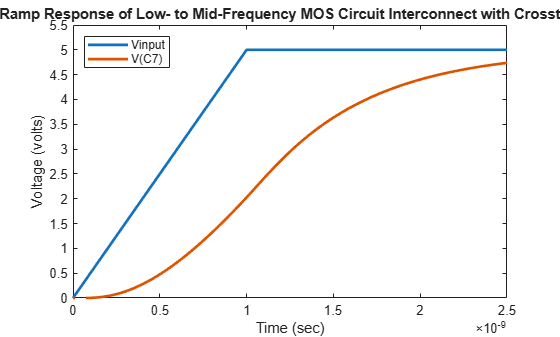Plot the crosstalk in low- to mid-frequency MOS circuit interconnect with ramp input.

```figure plot(t,input,t,outputC12,'LineWidth',2) axis([0 5e-9 0 .5]); title('Crosstalk in Low- to Mid-frequency MOS Circuit Interconnect with Ramp Input'); xlabel('Time (sec)'); ylabel('Voltage (volts)'); legend('Vinput','V(C12)','Location','NorthEast');```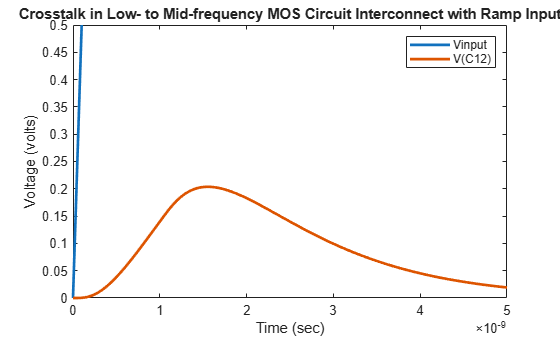### Verify Rational Fit Outside Fit Range

Though not shown in this example, you can also use the `freqresp` function to check the behavior of `rationalfit` function well outside the specified frequency range. The fit outside the specified range can sometimes cause surprising behavior, especially if frequency data near 0 Hz (DC) was not provided.

To perform this check for the rational-function approximation in this example, uncomment and run the following MATLAB code.

```% widerFreqs = logspace(0,12,1001); % respC7 = freqresp(fitC7,widerFreqs); % figure % loglog(freqs,abs(tfC7),'+',widerFreqs,abs(respC7)) % respC12 = freqresp(fitC12,widerFreqs); % figure % loglog(freqs,abs(tfC12),'+',widerFreqs,abs(respC12))```